Instant Maths Help:

# Edexcel A Level - Functions

## Functions, Composites, Inverses & Models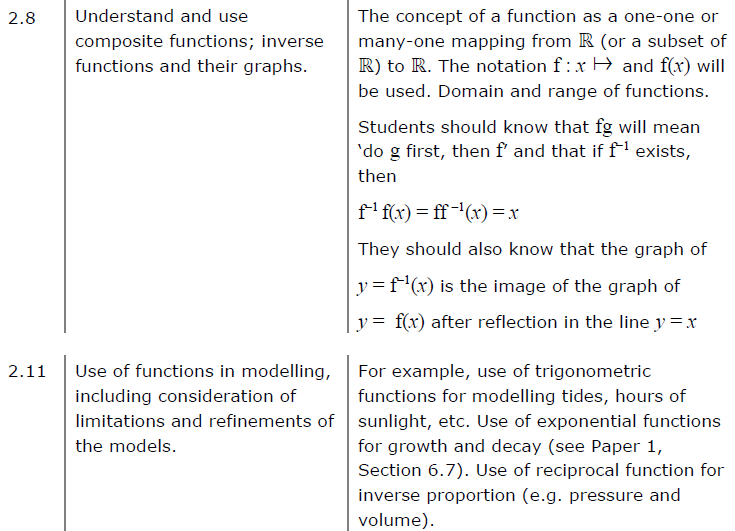##### Edexcel 9MA0/01 Jun 2023 A2 Exam Q. 7 :   8 marks in 9:36 min.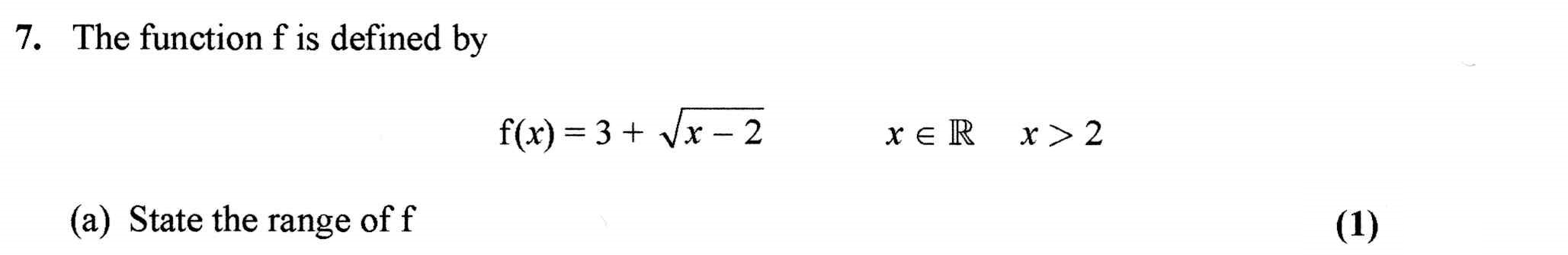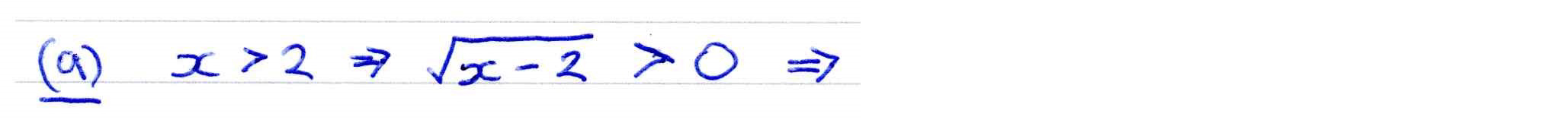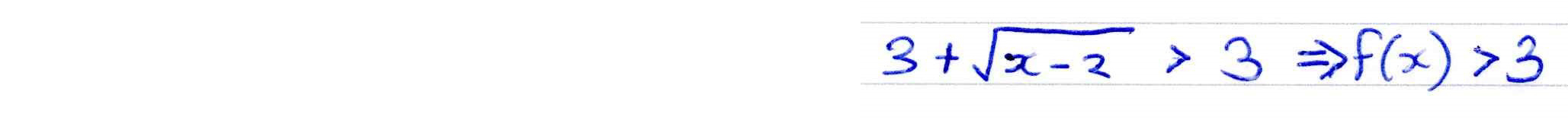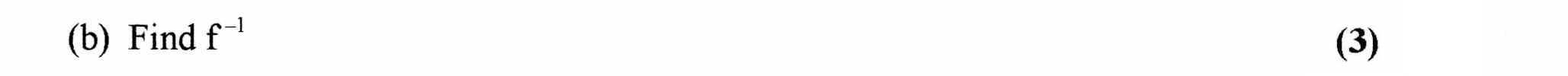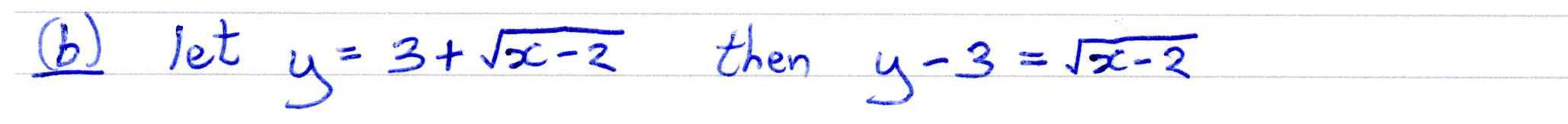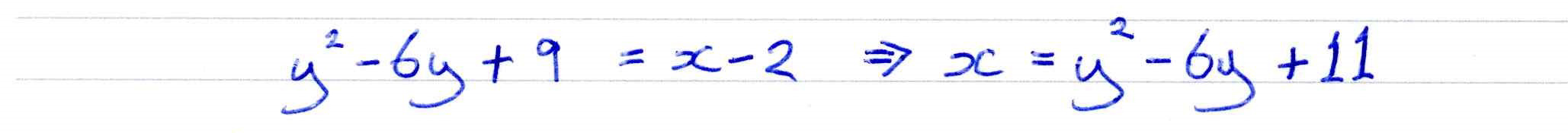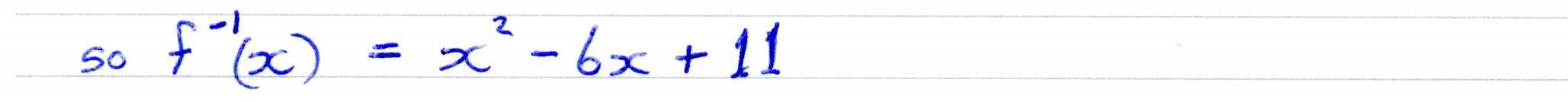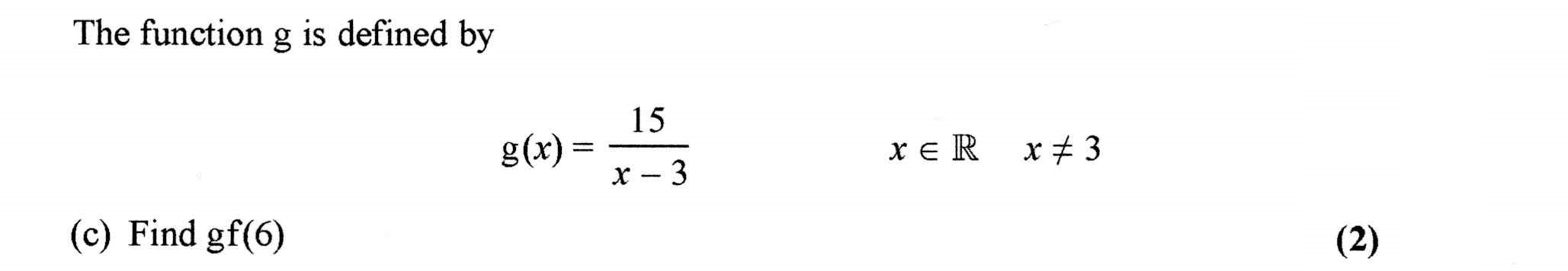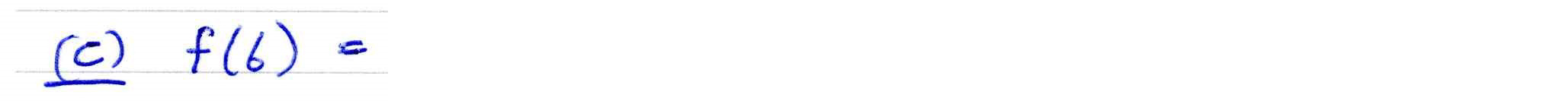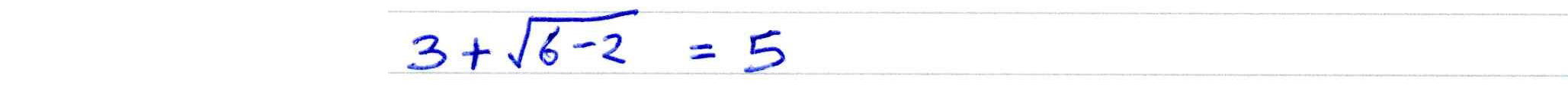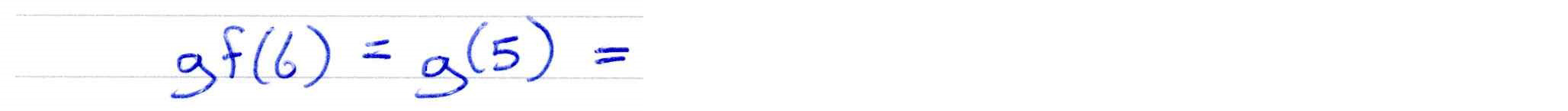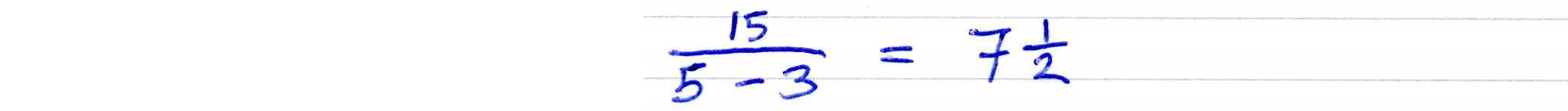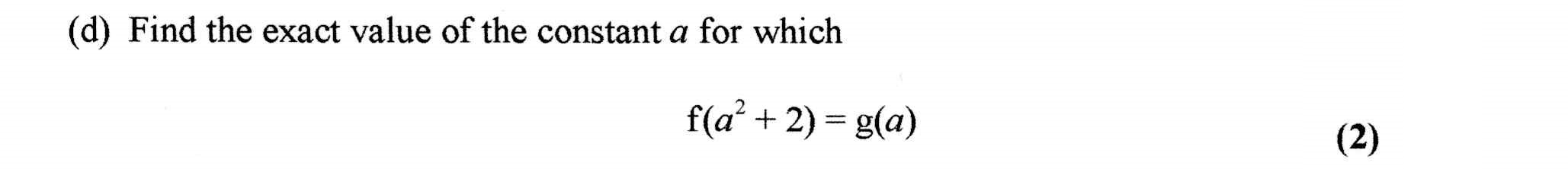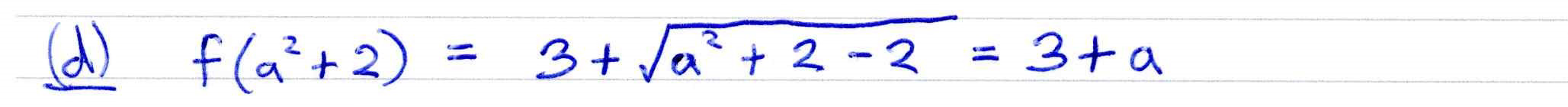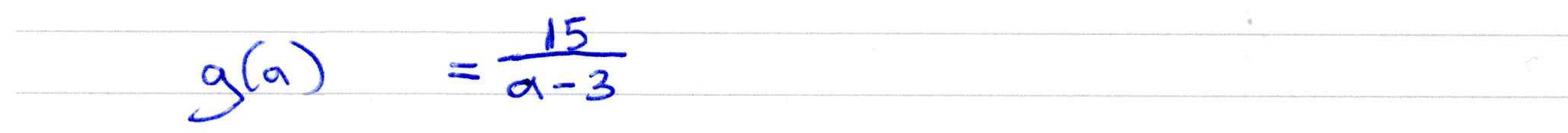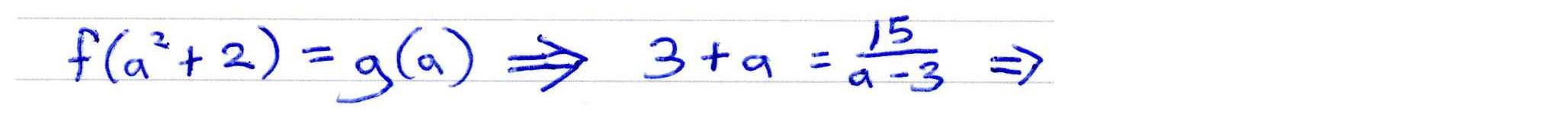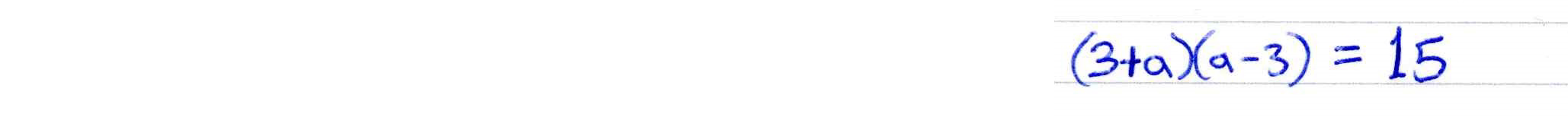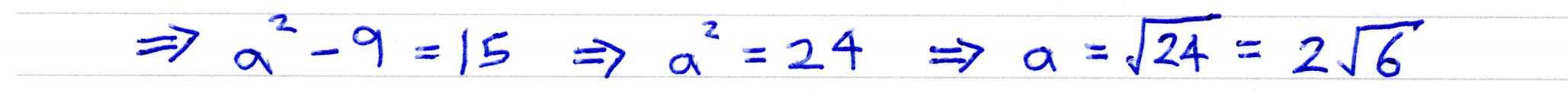##### Domain and Range of Function
The line $y = a{x^3} - x$ is displayed for the domain $d \le x \le e$.

Observe how the choice of domain affects the range.

##### Inverse Functions
Use this display to explore the composition of functions with their inverses. Move the drag point. If f(x) and g(x) are inverses then gf(x) should equal x. Try each of the 14 combinations provided. Why is it that sometimes gf(x) is not x?